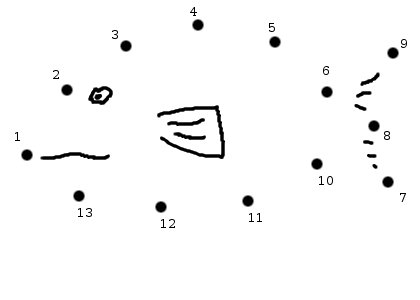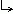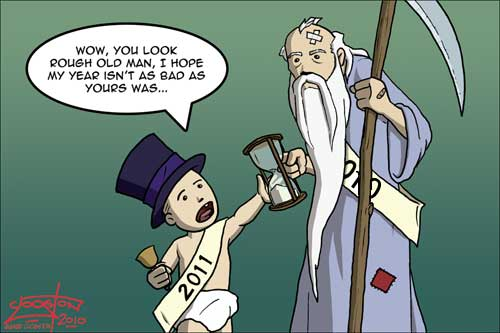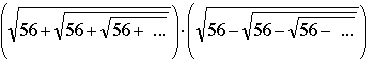Math Central - mathcentral.uregina.caProblem of the Month 2010-2011Currentproblem Recent problemswith solutions
 Older problems from 2005/2006 06/07 07/08 08/09 09/10 10/11 11/12

 PM105: May, 2011This month's problem investigates connect-the-dots puzzles. As a warm-up exercise you can complete the accompanying puzzle to discover an excellent source of vitamins. (Please do not submit your solution to the practice puzzle.)(Corrected.) Such a puzzle is a collection of $n$ distinct points in the plane numbered from $1$ to $n$ such that no line contains three consecutive points $i, i+1, i+2$ (or $n-1, n, 1$). Our problem this month comes in two parts. Show that for every $n>13$ you can produce a connect-the-dots puzzle with $n$ points such that every line of the plane that contains a pair of consecutive points will contain at least one other pair of consecutive points. Show that for any 13-point puzzle there exists a straight line that contains one and only one pair of consecutive points.Solution. pdf version. PM104: April, 2011Does there exist a continuous real-valued function $f: \mathbb{ R}\rightarrow \mathbb{ R}\;$ that takes each of its values exactly twice? Does there exist a continuous real-valued function $f: \mathbb{ R}\rightarrow \mathbb{ R}\;$ that takes each of its values exactly three times?Solution. pdf version. PM103: March 2011This month's problem is our tribute to Jorge Luis Borges, the author of The Book of Sands and The Library of Babel. The Cabbalistic Encyclopedia of Permutations of the Roman Alphabet consists of seven volumes listing the permutations of the 26-letter Roman alphabet in alphabetical order.  The volumes are very large, and written in very small print, because there are many of these permutations.  Each volume contains exactly one-seventh of all permutations; if you open Volume 1 at the very first page and peer through your magnifying glass, you will see the first permutation abcdefghijklmnopqrstuvwxyz appearing at the top, followed immediately by abcdefghijklmnopqrstuvwxzy. Our problem: What is the very last permutation listed in this first volume?Solution. pdf version. PM102: February 2011For which positive integers $n$ and $a_1, a_2, a_3, \dots, a_n$ is the product $a_1\cdot a_2 \cdot a_3 \cdot \dots \cdot a_n$ as large as possible when these numbers must satisfy $a_1 + a_2 + a_3 + \dots + a_n = 2011$?Solution. pdf version. PM101: January 2010Heron's formula tells us that the area of a triangle is determined by the lengths of its three sides; is it true that the volume of a tetrahedron is determined by the areas of its four faces? Our regular correspondents will notice that we changed the method used to display the mathematical expressions in the solution. This technique, which employs MathJax, requires the use of Javascript. For users who do not have Javascript installed we are including a pdf version of the solution. If you have difficulties with either format please let us know.Solution.pdf version. PM100: December 2010Is (20102011!)2 greater than 2010201120102011?Solution. PM99: November 2010Computeand justify your answer.Solution. PM98: October 2010Find all primes p such that 2p + p2 is also a prime.Solution. PM97: September 2010A plumber with a pile of equal-length straight pipes and a box full of right-angled elbows realizes that he cannot use them to form a closed pentagon, although he can make a closed polygon with six or with seven pipes. Prove that plumber's observations are indeed correct. (For those mathematicians with no training in plumbing we should add that the pipes cannot be joined directly together -- neighbouring pipes can be joined only by using an elbow, thereby forming a right angle.)Solution.
 Math Central is supported by the University of Regina and the Pacific Institute for the Mathematical Sciences.about math central :: site map :: links :: notre site français